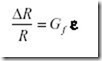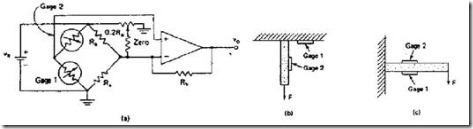" /> Strain Gauge Position Sensor | TN Industri
Home > English > Strain Gauge Position Sensor

# Strain Gauge Position Sensor

Position measurement can be done by analog and digital. For a shift that is not too far away measurements can be done using analog means, while for longer shift distance it is better to use digital way. Position or displacement sensor results can be used to measure linear or angular displacement. Technical treatment of the sensor can be done by directly connected (contact) and not connected directly (without contact).

Strain gauge can be used as a position sensor. SG in its operation exploits a change of resistance so that it can be used to measure the very small displacement due to tensile strains . The definition of elasticity ( ε ) strain gauge is the ratio of the length change (ΔL) to the original length ( L ), namely:or the ratio of resistance change ( R ) to the original resistance ( R ) is equal to the gage factor ( G f ) times the elasticity of starin gage ( ε ):SG construction is made of thin metal material (foil) placed on paper. For SG detection process attached with the specimen in two ways:
1. Direction of stretching / stretching made as long as possible (axial)
2. The direction of perpendicular density / stretching is made as short as possible (lateral)Figure 3.24 Physical form of strain gauge

The gauge factor ( G f ) represents the elasticity level of the metal material of the SG.
• incompressible metal Gf = 2
• piezoresistive Gf = 30 /
• piezoresistive sensors are used on pressure sensor ICs

To perform the sensor on the specimen the circuit and placement of SG is
• arranged in a series of bridges
• two strain gauges are used adjacent, one for stretching / density, one for temperature compensation at positions not affected by stretching / density
• frequency response is determined where the strain gauge is locatedFigure 3.25 Installation of strain gauge: (a) bridge circuit
(b) gage1 and gage 2 positions 90 (c) gage 1 and gage 2 parallel positions